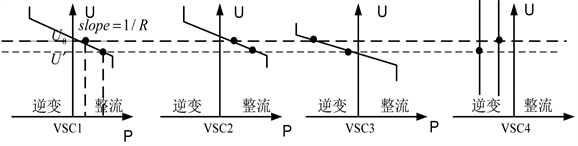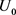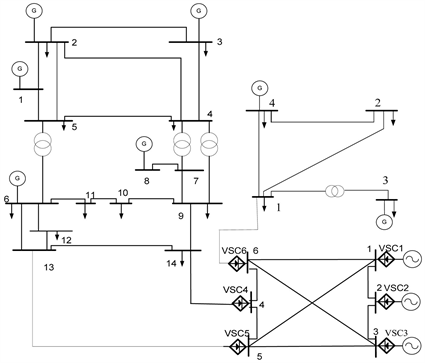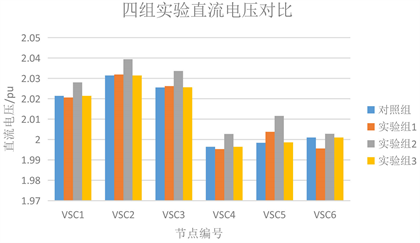# VSC电压下垂参数对交直流联合电网网损的影响Influence of VSC Voltage Droop Parameter on Network Loss of AC/DC Combined Power Grid

• 全文下载: PDF(1004KB)    PP.112-118   DOI: 10.12677/SG.2019.93012
• 下载量: 247  浏览量: 355

At present, as for AC/DC combined power grid, most of its DC voltage control methods use voltage droop control. In order to improve the system operation reliability and reduce the network loss under the droop control mode, this paper explores the influence of the controllable parameters of the VSC voltage droop control on the system network loss. Firstly, the AC-DC combined grid network loss calculation model considering the converter loss and the DC grid steady-state model under the VSC voltage droop control mode are established. The principle of VSC droop control and the influence of different parameters on the P-V characteristic curve are analyzed. Through the simulation of the example, the DC voltage fluctuation and the network loss optimization effect when adjusting the different control parameters of the converter are compared. Finally, it is concluded that the network loss optimization effect of adjusting the droop control reference power is good and can guarantee the safe and stable operation of the system. It is an effective reactive power optimization measure.

1. 引言

2. 交直流联合电网网损计算模型

${P}_{\text{loss}}={P}_{\text{ac}L}+{P}_{\text{dc}L}+{P}_{\text{vsc}}$ (1)

$\left\{\begin{array}{l}{P}_{\text{ac}L}=\underset{k=1}{\overset{{n}_{1}}{\sum }}{G}_{k\left(i,j\right)}\left({U}_{i}^{2}+{U}_{j}^{2}-2{U}_{i}{U}_{j}\mathrm{cos}\left({\theta }_{i}-{\theta }_{j}\right)\right)\\ {P}_{\text{dc}L}=\underset{k=1}{\overset{{n}_{2}}{\sum }}{I}_{\text{d}k}^{2}{R}_{\text{d}k}\\ {P}_{\text{vsc}}=\underset{i=1}{\overset{{n}_{T}}{\sum }}{P}_{\text{vsc}i}=\underset{i=1}{\overset{{n}_{T}}{\sum }}\left({a}_{i}{I}_{\text{c}i}^{2}+{b}_{i}{I}_{\text{c}i}+{c}_{i}\right)\end{array}$ (2)

3. 交直流联合电网直流电压控制方式

3.1. 直流电压控制方式分类

3.2. 电压下垂控制原理Figure 1. Principle of voltage droop control

${P}_{\text{d}i}={P}_{0i}+{K}_{i}\left({U}_{0i}-{U}_{\text{d}i}\right)$ (3)

VSC4采用定功率控制，当VSC4出现功率波动时，换流站的工作电压均由变为 ${U}^{\prime }$ ，VSC1、VSC2、VSC3会沿着各自的P-V特性曲线寻找新的工作点，从而使系统重新回到功率平衡。多个换流站共同承担维持系统功率平衡的任务，增加了系统的运行可靠性。

3.3. 电压下垂控制下的直流电网模型

${I}_{\text{d}i}=\frac{{P}_{\text{d}i}}{{U}_{\text{d}i}}=\underset{j=1}{\overset{{n}_{\text{vsc}}}{\sum }}{G}_{ij}{U}_{\text{d}j}$ (4)

$\left[\begin{array}{c}\frac{{P}_{01}+{K}_{1}\left({U}_{01}-{U}_{\text{d}1}\right)}{{U}_{\text{d}1}}\\ \frac{{P}_{02}+{K}_{2}\left({U}_{02}-{U}_{\text{d}2}\right)}{{U}_{\text{d}2}}\\ ⋮\\ \frac{{P}_{0i}+{K}_{i}\left({U}_{0i}-{U}_{\text{d}i}\right)}{{U}_{\text{d}i}}\end{array}\right]=\left[\begin{array}{cccc}{G}_{11}& {G}_{12}& \cdots & {G}_{12}\\ {G}_{12}& {G}_{22}& \cdots & {G}_{12}\\ ⋮& ⋮& \ddots & ⋮\\ {G}_{1n}& {G}_{12}& \cdots & {G}_{12}\end{array}\right]\left[\begin{array}{c}{U}_{\text{d}1}\\ {U}_{\text{d}2}\\ ⋮\\ {U}_{\text{d}i}\end{array}\right]$ (5)

4. 算例分析

4.1. 仿真条件Figure 2. Topology of the example systemTable 1. Constraints on generator outputTable 2. Comparison of droop control parameters of VSC5 and VSC6 in four groups of experiments

4.2. 仿真结果分析

1) 直流电压对比Figure 3. DC voltage comparison before and after optimization of scenes 1, 2 and 3

2) 系统网损优化效果对比

VSC不同参数的网损优化效果如表3所示，由图表数据可知，优化VSC5、VSC6下垂控制参考功率或参考电压，其输出功率发生显著变化，系统网损也大大降低；而对换流器电压下垂系数来说，即使将其调至最优也不能使换流器输出功率发生明显变化，系统网损优化效果不佳。Table 3. Comparison of network loss optimization effects of different parameters of VSC

5. 结论

  温家良, 吴锐, 彭畅, 等. 直流电网在中国的应用前景分析[J]. 中国电机工程学报, 2012, 32(13): 7-12.  汤广福, 罗湘, 魏晓光. 多端直流输电与直流电网技术[J]. 中国电机工程学报, 2013, 33(10): 8-17.  梁旭明, 张平, 常勇. 高压直流输电技术现状及发展前景[J]. 电网技术, 2012, 36(4): 1-9.  赵成勇, 郭春义, 刘文静. 混合直流输电系统[M]. 北京: 科学出版社, 2014.  张众. 交流/直流联合电网稳态分析方法研究[D]: [硕士学位论文]. 哈尔滨: 哈尔滨工业大学, 2015.  邱迪. 交流/混合直流联合系统最优潮流分析[D]: [硕士学位论文]. 哈尔滨: 哈尔滨工业大学, 2017.  孙黎霞, 陈宇, 宋洪刚, 等. 适用于VSC-MTDC的改进直流电压下垂控制策略[J]. 电网技术, 2016, 40(4): 1037-1043.  李子寿, 周密, 徐箭, 等. 考虑交流电网调节能力的VSC-MTDC改进下垂控制方法[J]. 电力建设, 2016, 37(6): 79-85.  王渝红, 陈勇, 曾琦, 等. 适用于VSC-MTDC的改进下垂控制[J]. 高电压技术, 2018, 44(10): 3190-3196.  和敬涵, 李智诚, 王小君, 等. 计及换流器损耗与电压下垂控制的交直流系统最优潮流算法[J]. 电力系统及其自动化, 2017, 41(22): 48-55.  Daelemans, G. (2008) VSC HVDC in Meshed Networks. Catholic University of Louvain, Belgium.  李程昊, 谢竹君, 林卫星, 等. 中高频模块化多电平换流器阀损耗的精确计算方法与分析平台[J]. 中国电机工程学报, 2015, 35(17): 4361-4370.  江全元, 耿光超. 含高压直流输电系统的内点法最优潮流算法[J]. 中国电机工程学报, 2009, 29(25): 43-44.  耿光超, 江全元. 基于自动微分技术的内点法最优潮流算法[J]. 电力系统及其自动化, 2008, 32(23): 41-45.  覃智君, 阳育德, 吴杰康. 矢量化动态最优潮流计算的步长控制内点法实现[J]. 中国电机工程学报, 2009, 29(7): 52-58.